Main Content

# spiralRectangular

Create rectangular spiral antenna on X-Y plane

## Description

The `spiralRectangular` object creates a single or two-arm rectangular spiral antenna. The default rectangular spiral has two arms, is center-fed and is on the X-Y plane. The default resonating frequency is 7.65 GHz.

A spiral rectangular antenna is made up of filaments. The distance between the two violet dashed lines in the diagram represents the first filament or the initial width. The distance between the two orange dashed lines in the diagram represents the second filament or the initial length.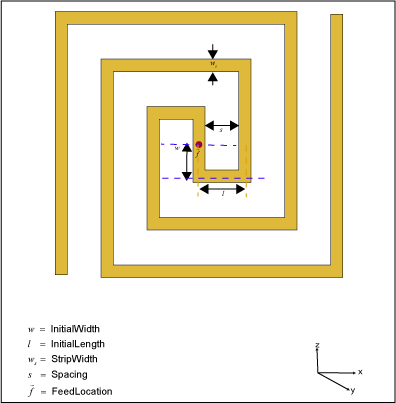## Creation

### Syntax

``ant = spiralRectangular``
``ant = spiralRectangular(Name,Value)``

### Description

example

````ant = spiralRectangular` creates a default rectangular spiral antenna object operating at 7.65 GHz.```
````ant = spiralRectangular(Name,Value)` sets properties using one or more name-value pairs. For example, `ant = spiralRectangular('NumArms',1)` creates a rectangular spiral antenna object with one arm. Enclose each property name in quotes.```

## Properties

expand all

Number of arms of the spiral, specified as `1` or `2`.

Example: `'NumArms',1`

Example: `ant.NumArms = 1`

Data Types: `double`

Number of turns in the spiral, specified as a scalar in meters. One turn length is taken as the length of a complete 360-degree revolution. To calculate the length of 1.25 turns, the first spiral is created up to one turn. Then the length of the second turn is scaled to the given fraction and added to the first turn length.

Example: `'NumTurns',2.0`

Example: `ant.NumTurns = 2.0`

Data Types: `double`

Length of the first filament along the Y-axis from the origin to the midline of the strip width of the second filament, specified as a scalar in meters. `InitialWidth` is the width between the dashed violet color lines in the antenna image.

Example: `'InitialWidth',0.0050`

Example: `ant.InitialWidth = 0.0050`

Data Types: `double`

Length of the second filament along the X-axis from the mid line of the first filament to half of the strip width of the third filament, specified as a scalar in meters. `InitialLength` is the width between the dashed orange color lines in the antenna image.

Example: `'InitialLength',0.0055`

Example: `ant.InitialLength = 0.0055`

Data Types: `double`

Width of the strip, specified as a scalar in meters.

Example: `'StripWidth',5.0050e-04`

Example: `ant.StripWidth = 5.0050e-04`

Data Types: `double`

Spacing between turns of the spiral, specified as a scalar in meters.

Example: `'Spacing',0.0015`

Example: `ant.Spacing = 0.0015`

Data Types: `double`

Direction of the spiral turns (windings), specified as `'CW'` or `'CCW'`.

Example: `'WindingDirection'`,`'CW'`

Example: `ant.WindingDirection = CW`

Data Types: `char` | `string`

Type of the metal used as a conductor, specified as a metal material object. You can choose any metal from the `MetalCatalog` or specify a metal of your choice. For more information, see `metal`. For more information on metal conductor meshing, see Meshing.

Example: ```m = metal('Copper'); 'Conductor',m```

Example: ```m = metal('Copper'); ant.Conductor = m```

Lumped elements added to the antenna feed, specified as a `lumpedElement` object. You can add a load anywhere on the surface of the antenna. By default, the load is at the feed. For more information, see `lumpedElement`.

Example: `'Load',lumpedElement`, where `lumpedElement` is load added to the antenna feed.

Example: `ant.Load = lumpedElement('Impedance',75)`

Tilt angle of the antenna, specified as a scalar or vector with each element unit in degrees. For more information, see Rotate Antennas and Arrays.

Example: `'Tilt',90`

Example: `ant.Tilt = 90`

Example: `'Tilt',[90 90]`,`'TiltAxis',[0 1 0;0 1 1]` tilts the antenna at 90 degrees about the two axes defined by the vectors.

Note

The `wireStack` antenna object only accepts the dot method to change its properties.

Data Types: `double`

Tilt axis of the antenna, specified as:

• Three-element vector of Cartesian coordinates in meters. In this case, each coordinate in the vector starts at the origin and lies along the specified points on the X-, Y-, and Z-axes.

• Two points in space, each specified as three-element vectors of Cartesian coordinates. In this case, the antenna rotates around the line joining the two points in space.

• A string input describing simple rotations around one of the principal axes, 'X', 'Y', or 'Z'.

For more information, see Rotate Antennas and Arrays.

Example: `'TiltAxis',[0 1 0]`

Example: `'TiltAxis',[0 0 0;0 1 0]`

Example: `ant.TiltAxis = 'Z'`

Note

The `wireStack` antenna object only accepts the dot method to change its properties.

Data Types: `double`

## Object Functions

 `show` Display antenna or array structure; display shape as filled patch `axialRatio` Axial ratio of antenna `beamwidth` Beamwidth of antenna `charge` Charge distribution on metal or dielectric antenna or array surface `current` Current distribution on metal or dielectric antenna or array surface `design` Design prototype antenna or arrays for resonance at specified frequency `efficiency` Radiation efficiency of antenna `EHfields` Electric and magnetic fields of antennas; Embedded electric and magnetic fields of antenna element in arrays `impedance` Input impedance of antenna; scan impedance of array `mesh` Mesh properties of metal or dielectric antenna or array structure `meshconfig` Change mesh mode of antenna structure `optimize` Optimize antenna or array using SADEA optimizer `pattern` Radiation pattern and phase of antenna or array; Embedded pattern of antenna element in array `patternAzimuth` Azimuth pattern of antenna or array `patternElevation` Elevation pattern of antenna or array `returnLoss` Return loss of antenna; scan return loss of array `sparameters` S-parameter object `vswr` Voltage standing wave ratio of antenna `rectspirallength2turns` Calculate number of turns for specified arm length in rectangular spiral antenna

## Examples

collapse all

Create and view a default rectangular spiral antenna.

`ant = spiralRectangular`
```ant = spiralRectangular with properties: NumArms: 2 NumTurns: 1.5300 InitialWidth: 0.0010 InitialLength: 0.0015 StripWidth: 4.0500e-04 Spacing: 0.0011 WindingDirection: 'CCW' Conductor: [1x1 metal] Tilt: 0 TiltAxis: [1 0 0] Load: [1x1 lumpedElement] ```
`show(ant)`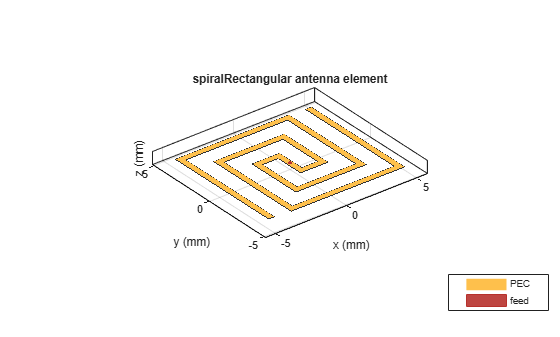Plot the radiation pattern of the antenna at the default frequency.

`pattern(ant,7.65e9)`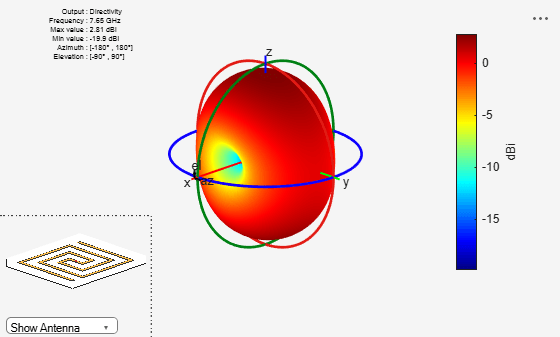Create a rectangular spiral antenna object with two arms and two turns.

```ant_d = spiralRectangular('NumArms',2,'NumTurns',2,'InitialLength',1e-3,... 'InitialWidth',1e-3,'Spacing',0.5e-3,'StripWidth',0.5e-3);```

Back the spiral using a reflector antenna object.

``` r = reflector('Exciter',ant_d,'GroundPlaneLength',15e-3,'GroundPlaneWidth',... 15e-3,'Spacing',2e-3,'Substrate',dielectric('FR4')); figure; show(r);```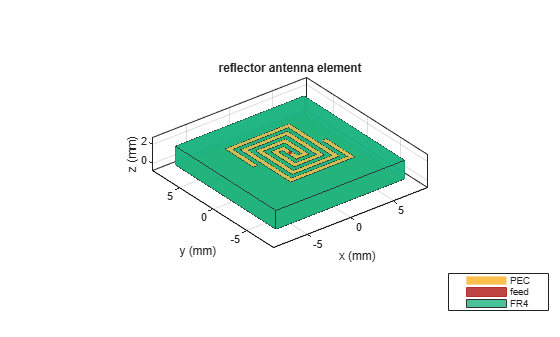Plot the radiation pattern of the antenna at the specified frequency.

``` figure; pattern(r,8e9);```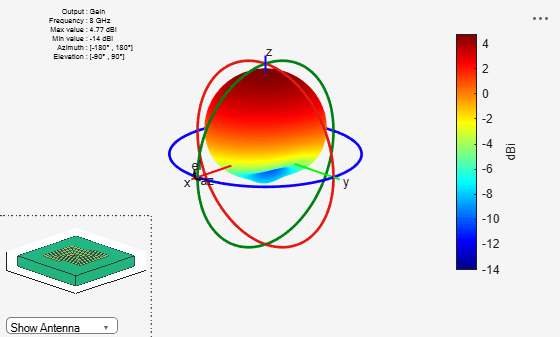Create a single arm rectangular spiral antenna with a total arm length of 291 mm.

```ant = spiralRectangular('NumArms',1,'NumTurns',3,'InitialLength',4.5e-3,... 'InitialWidth',4.5e-3,'Spacing',3.3e-3,'StripWidth',1.2e-3); nT = rectspirallength2turns(ant,291e-3); ant.NumTurns = nT; figure; show(ant); ```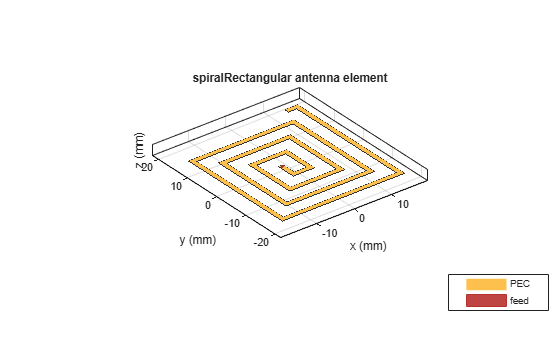Nakano, H., H. Yasui, and J. Yamauchi. “Numerical Analysis of Two-Arm Spiral Antennas Printed on a Finite-Size Dielectric Substrate.” IEEE Transactions on Antennas and Propagation 50, no. 3 (March 2002): 362–70. https://doi.org/10.1109/8.999628.

 Nakano, H., J. Eto, Y. Okabe, and J. Yamauchi. “Tilted- and Axial-Beam Formation by a Single-Arm Rectangular Spiral Antenna with Compact Dielectric Substrate and Conducting Plane.” IEEE Transactions on Antennas and Propagation 50, no. 1 (January 2002): 17–24. https://doi.org/10.1109/8.992557.

## Support

#### Hybrid Beamforming for Massive MIMO Phased Array Systems

Download the white paper